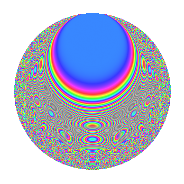# Properties

 Label 2009.2.cLevel 2009 Weight 2 Character orbit c Rep. character $$\chi_{2009}(491,\cdot)$$ Character field $$\Q$$ Dimension 138 Sturm bound 392

# Related objects

## Defining parameters

 Level: $$N$$ = $$2009 = 7^{2} \cdot 41$$ Weight: $$k$$ = $$2$$ Character orbit: $$[\chi]$$ = 2009.c (of order $$2$$ and degree $$1$$) Character conductor: $$\operatorname{cond}(\chi)$$ = $$41$$ Character field: $$\Q$$ Sturm bound: $$392$$

## Dimensions

The following table gives the dimensions of various subspaces of $$M_{2}(2009, [\chi])$$.

Total New Old
Modular forms 204 148 56
Cusp forms 188 138 50
Eisenstein series 16 10 6

## Trace form

 $$138q + 2q^{2} + 134q^{4} + 4q^{5} - 6q^{8} - 126q^{9} + O(q^{10})$$ $$138q + 2q^{2} + 134q^{4} + 4q^{5} - 6q^{8} - 126q^{9} + 134q^{16} - 14q^{18} + 24q^{20} - 16q^{23} + 98q^{25} + 8q^{31} + 30q^{32} - 158q^{36} + 20q^{37} + 4q^{39} + 8q^{40} + 12q^{41} + 24q^{43} - 36q^{45} - 24q^{46} - 70q^{50} + 24q^{51} - 8q^{57} + 28q^{59} - 20q^{61} - 40q^{62} + 78q^{64} - 72q^{66} + 30q^{72} + 36q^{73} - 24q^{74} + 16q^{78} + 40q^{80} + 82q^{81} - 24q^{82} - 16q^{83} - 56q^{86} - 4q^{87} + 76q^{90} - 40q^{92} + O(q^{100})$$

## Decomposition of $$S_{2}^{\mathrm{new}}(2009, [\chi])$$ into irreducible Hecke orbits

The newforms in this space have not yet been added to the LMFDB.

## Decomposition of $$S_{2}^{\mathrm{old}}(2009, [\chi])$$ into lower level spaces

$$S_{2}^{\mathrm{old}}(2009, [\chi]) \cong$$ $$S_{2}^{\mathrm{new}}(41, [\chi])$$$$^{\oplus 3}$$$$\oplus$$$$S_{2}^{\mathrm{new}}(287, [\chi])$$$$^{\oplus 2}$$